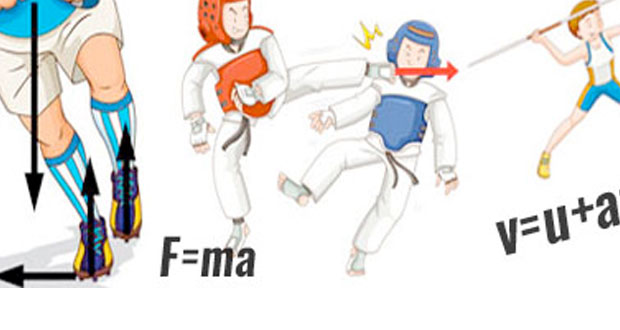# Biomechanics

Biomechanics is the mathematics of technique. An understanding of biomechanics enables you to understand the perfect technique of just about any sporting activity. Here we explain in simple terms the laws of physics as they relate to sport.

## Linear Motion

Linear motion simply means motion in a straight line (as opposed to circular motion or rotation). In order to talk about linear motion scientifically, we need to be familiar with mass, distance, displacement, speed, velocity, and acceleration. Here we explain Newton’s laws of motion, mass, inertia, momentum, speed, velocity, distance, displacement and graphs of motion.

## Forces

A force is a push or a pull which alters the state of motion of a body and is measured in Newtons (N). Here we cover balanced and unbalanced forces, friction, air resistance, impulse, force-time graphs, and free body diagrams.

## Angular Motion

Angular momentum includes rotating bodies, levers, stability, moment of force/torque, axis of rotation, moment of inertia and angular momentum.

## Fluid Mechanics

Fluid mechanics or fluid dynamics comes into sport a lot and covers air resistance, drag, projectiles, spin on balls and Bernoulli principle and lift force.